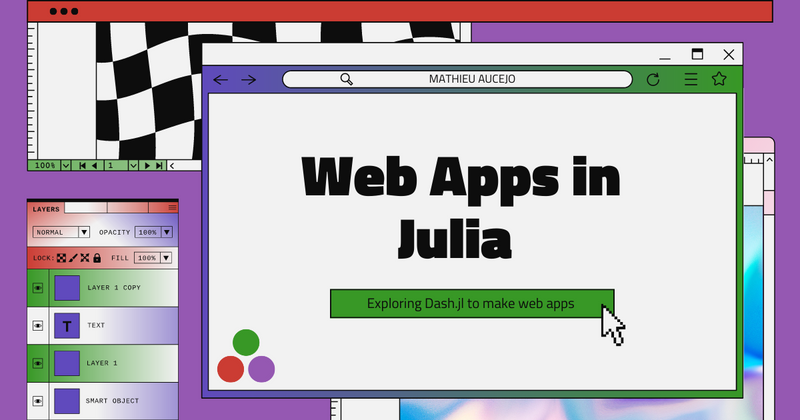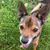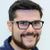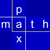## Julia Community 🟣Mathieu Aucejo

Posted on • Updated on

# Make beautiful web apps with... Dash.jl

Until recently, I mainly used Matlab and Python for my research and teaching activities. In the last context, I developed some apps to illustrate some concepts of mechanical vibrations such as the free vibration motion of undamped/damped spring-mass oscillators. To this end, my worflow was as follows:

1. Develop the apps using Ploty Dash in Python
2. Embed the apps in a reveal.js presentation using HTML iframe

But all of this was before I decided to move from Matlab/Python to Julia. As every Julian newcomer, I had to revise my workflow, find the right packages, think the Julian way, and so on. Everything is not perfect, but I like coding in Julia and the community is really supportive with newbies. That is why, I decided to translate my Dash.py apps into Dash.jl apps.

## 1. First steps

When I want to discover a framework, I directly go to the documentation page or the dedicated website. Here, we have to visit the Plotly Dash website.

Good news! There is a Julia section. Here is a brief and non exhaustive list of what is dedicated to Julia:

1. How to install Dash.jl ?
2. Basic Dash layout. This section is ideal to start with Dash and develop static apps.
3. Basic callbacks or how to transform your Dash layout into an interactive app.
4. A section dedicated to advanced callbacks.

And that's all for the moment (Dec. 2022), since the other parts of the doc are either populated with Python examples or simply not written. For instance, in the interactive visualizations section, it is indicated:

This example has not been ported to Julia yet - showing the Python version instead.

Visit the old docs site for Julia at: https://community.plotly.com/c/dash/julia/20

In the other sections, it is mentioned:

This page is not yet available in Julia — check back soon! In the meantime, you can refer to the Python documentation on this topic or post your question in the Dash Julia forum!

Humm! It is not very engaging... But, Plotly people are right! Indeed, even if everything is not documented, the available documentation combined with the Python one is sufficient to to develop our superb web apps in Julia!

## 2. A little maths

The equation of motion of a damped spring-mass system is given by:

$\ddot x(t) + 2\xi\omega_0\dot x(t) + \omega_0^2 = 0,$

where $x(t)$ is the displacement of the mass, $\xi$ is the damping ratio and $\omega_0$ is the natural angular frequency.

Depending on the value of the daming ratio, we can observe four distinct behavior for a given set of initial displacement $x_0$ and velocity $v_0$ .

• If $\xi = 0$ , the motion of the mass is undamped and:
$x(t) = x_0\cos\omega_0 t + \frac{v_0}{\omega_0}\sin\omega_0 t,$
• if $\xi \in ]0, 1[$ , the motion of the mass is under-damped and:
$x(t) = \left[x_0\cos\Omega_0 t + \frac{v_0 + \xi\omega_0 x_0}{\Omega_0}\sin\Omega_0 t\right]e^{-\xi\omega_0 t},$
where $\Omega_0 = \omega_0\sqrt{1 - \xi^2}$ is the pseudo natural angular frequency.
• if $\xi > 1$ , the motion of the mass is over-damped and:
$x(t) = \left[x_0\cos\beta t + \frac{v_0 + \xi\omega_0 x_0}{\Omega_0}\sin\beta t\right]e^{-\xi\omega_0 t}$
where $\beta = \omega_0\sqrt{\xi^2 - 1}.$
• if where $\xi = 1$ , the motion of the mass is critically damped and: where
$x(t) = (x_0 + \omega_0 x_0 t + v_0 t)e^{-\omega_0 t}$
.

The goal of the app is to plot the displacement of the mass for a given set where $(f_0, \xi, x_0, v_0)$ , where $f_0 = \frac{\omega_0}{2\pi}$ is the resonance frequency of the mass-spring system. More precisely, we want to obtain something that looks like the following picture

## 3. Let's code this in Julia using Dash.jl

To make things clearer, we will follow a step-by-step approach.

### Computation of the free vibration response

In this part, we want to code the equations describing the free vibration motion of the mass-spring system. It is basically a trivial task in Julia.

const t = 0.:1e-3:2.

function response(f₀ = 10., ξ = 0.01, x₀ = 1., v₀ = 1.)
ω₀ = 2π*f₀     # Natural angular frequency
if 0. ≤ ξ < 1. # Undamped/Under-damped response
Ω₀ = ω₀*√(1. - ξ^2)
x = (x₀*cos.(Ω₀*t) + (v₀ + ξ*ω₀*x₀)*sin.(Ω₀*t)/Ω₀).*exp.(-ξ*ω₀*t)
elseif ξ == 1.  # Critically damped response
x = @. (x₀ + ω₀*x₀*t + v₀*t)*exp(-ω₀*t)
else # Over-damped response
β = ω₀*√(ξ^2 - 1.)
x = @. (x₀*cosh(β*t) + (v₀ + ξ*ω₀*x₀*sinh(β*t))/β)*exp(-ξ*ω₀*t)
end
end


Just a comment here. The time variable t is defined as a global variable to avoid defining it each time the application is updated.

### Definition of the UI

The UI of our app contains:

• A plot
• Four sliders

To implement our UI, we have use Dash.jl and PlotlyJS.jl packages and define a custom css to define the UI layout.

Let's first implement our custom css. The app.css file is created in a folder named assets in the root folder of our app. For the present app, the css file is defined below.

/* /assets/app.css */

html {
font-size: 62.5%; }
body {
font-size: 1.5em;
font-family: "Open Sans";
color: rgb(50, 50, 50); }

h1 {
font-size: 3em;
}

.container {
position: relative;
width: 100%;
max-width: 960px;
margin: 0 auto;
box-sizing: border-box; }
.container:after,
.row:after,
.column,
.columns {
width: 100%;
float: left;
box-sizing: border-box; }

/* Media Queries
–––––––––––––––––––––––––––––––––––––––––––––––––– */
/* Larger than mobile */
@media (min-width: 400px) {}

/* Larger than phablet (also point when grid becomes active) */
@media (min-width: 550px) {}

/* Larger than tablet */
@media (min-width: 750px) {}

/* Larger than desktop */
@media (min-width: 1000px) {}

/* Larger than Desktop HD */
@media (min-width: 1200px) {}

/* Custom */
.modebar { top: 75px !important; }

/* For devices larger than 400px */
@media (min-width: 400px) {
.container {
width: 85%;
}

/* For devices larger than 550px */
@media (min-width: 550px) {
.container {
width: 80%; }
.column,
.columns {
margin-left: 0.5%; }
.column:first-child,
.columns:first-child {
margin-left: 0; }

.three.columns                  {width: 22%;}
.nine.columns                   {width: 74.0%;}
.offset-by-three.column,
.offset-by-three.columns        {margin-left: 26%;}
.offset-by-nine.column,
.offset-by-nine.columns         {margin-left: 78.0%;}
}


Now, we can design the application layout as depicted in the following figure.

Using Dash.jl, the previous layout can be implemented as follows

using PlotlyJS
using Dash

# Paste the code to compute the vibration response here

app = dash(external_stylesheets = ["/assets/app.css"])
app.title = "Free response of a mass-spring system"
app.layout = html_div() do
html_h1("Free response of a mass-spring system", style = Dict("margin-top" => 50)),

html_div(className = "row") do
html_div(className = "nine columns",
dcc_graph(
id = "graphic",
animate = true,
)
),

html_div(style = Dict("border" => "0.5px solid", "border-radius" => 5, "margin-top" => 68), className = "three columns") do
html_div(id = "freq-val",
style = Dict("margin-top" => "15px", "margin-left" => "15px", "margin-bottom" => "5px")),
dcc_slider(
id = "freq-slider",
min = 1.,
max = 50.,
step = 1,
value = 10.,
marks = Dict([i => ("$i") for i in [1, 10, 20, 30, 40, 50]]) ), html_div(id = "damp-val", style = Dict("margin-top" => "15px", "margin-left" => "15px", "margin-bottom" => "5px")), dcc_slider( id = "damp-slider", min = 1, max = 6, step = nothing, value = 1, marks = Dict([i => ("$(damping(i))") for i in 1:6])
),

html_div(id = "disp-val",
style = Dict("margin-top" => "15px", "margin-left" => "15px", "margin-bottom" => "5px")),
dcc_slider(
id = "disp-slider",
min = -1.,
max = 1.,
step = 0.1,
value = 0.5,
marks = Dict([i => ("$i") for i in [-1, 0, 1]]) ), html_div(id = "vel-val", style = Dict("margin-top" => "15px", "margin-left" => "15px", "margin-bottom" => "5px")), dcc_slider( id = "vel-slider", min = -100., max = 100., step = 1., value = 0., marks = Dict([i => ("$i") for i in [-100, -50, 0, 50, 100]])
)
end
end
end


• The app structure using the custom css is created from the command dash(external_stylesheets = ["/path/to/your/custom/css.css"]). The type of app is Dash.DashApp.
• The title appearing in the web browser tab is created from the command app.title = "Your incredible title".
• The layout of our app is defined in the app.layout, whose type is Component.
• The components html_xxx can be customized by using the keyword style, which is dictionary. The keys are the standard HTML properties (such as "margin-top", "border", ...). Hence, the HTML command style = "margin-top:10px; "border:1px solid" must be implemented as style = Dict("margin-top => "10px", "border" => "1px solid").
• Custom classes are defined by the keyword className.
• A specific id is given to each Dash components, in order to implement the callbacks making the app interactive.
• The slider components are encapsulated in a div environment having a specific id (e.g. freq-val), in order to define its title and display the current value of the slider.
• The damp-slider uses a set of discrete non-linearly spaced values. In order to make the gap between each value constant (it is a matter of taste), one has to define a mapping function damping. This function is implemented as follows:
function damping(value)
if value == 1
return 0.
elseif value == 2
return 0.001
elseif value == 3
return 0.01
elseif value == 4
return 0.1
elseif value == 5
return 1.
else
return 1.5
end
end


To this end, it is necessary to implement the so-called callbacks. A callback has one Output and one or several Input that apply to our app and the related Component. Here, one has to define 5 callbacks: 4 to define the titles of the sliders and 1 to update the graph.

The first four callbacks are easy to implement and are defined as follows:

callback!(
app,
Output("freq-val", "children"),
Input("freq-slider", "value")
) do freq_val
"Resonance frequency : $(freq_val) Hz" end callback!( app, Output("damp-val", "children"), Input("damp-slider", "value") ) do damp_val "Damping ratio :$(damping(damp_val))"
end

callback!(
app,
Output("disp-val", "children"),
Input("disp-slider", "value")
) do disp_val
"Initial displacement : $(disp_val) m" end callback!( app, Output("vel-val", "children"), Input("vel-slider", "value") ) do vit_val "Initial velocity :$(vit_val) m/s"
end


The last callback is a little bit more complex, since it has 4 inputs, corresponding to the outputs of the sliders. This callback implement the plotting function and the plot layout. This part is almost a 1:1 translation of the Python documentation.

callback!(
app,
Output("graphic", "figure"),
Input("freq-slider", "value"),
Input("damp-slider", "value"),
Input("disp-slider", "value"),
Input("vel-slider", "value")
) do f₀, ξ, x₀, v₀
rep = response(f₀, damping(ξ), x₀, v₀)
figure = (
data = [(
x = t,
y = rep,
type = "line",
hoverlabel = Dict(
"font" => Dict(
"size" => 14
)
)
)
],
layout =(
xaxis = Dict(
"title" => "Time (s)",
"titlefont" => Dict(
"size" => 20
),
"tickfont" => Dict(
"size" => 14
),
),
yaxis = Dict(
"title" => "Displacement (m)",
"titlefont" => Dict(
"size" => 20
),
"tickfont" => Dict(
"size" => 14
),
"range" => [minimum(rep), maximum(rep)],
"ticks" => "outside",
"tickcolor" => "white",
"ticklen" => 10
),
)
)
end


### Run the app

To run the app in a web browser, one has to use the run_server command with an optional port number (here 8050).

run_server(app, "0.0.0.0", 8050)


To see the result and play with the ap, you just have to visit the following address http://127.0.0.1:8050/ and voila. If you want to run your app directly without execute your app in the REPL, it is enough to execute in your terminal julia MyApp.jl.

## 4. Final thoughts

If you already know HTML and Python Dash, Dash.jl is a natural choice to build your web apps in Julia. If not, I hope this tutorial will help to convince you that Dash.jl is quite easy to use. However, I think that Genie Builder with its no-code VScode extension can be a game changer and I plan to make the app described in this tutorial with Genie to fairly compare both frameworks.

## Version info

Julia Version 1.8.3
Commit 0434deb161 (2022-11-14 20:14 UTC)
Platform Info:
OS: Windows (x86_64-w64-mingw32)
CPU: 8 × Intel(R) Core(TM) i7-10610U CPU @ 1.80GHz
WORD_SIZE: 64
LIBM: libopenlibm
LLVM: libLLVM-13.0.1 (ORCJIT, skylake)
Threads: 1 on 8 virtual cores

[1b08a953] Dash v1.1.2
[f0f68f2c] PlotlyJS v0.18.10Thank you for taking the time to write this tutorial!

I’m not so good with html/css. Would it have been possible to use the css file instead of the style keyword arguments for the html components?

Encore merci!

Edit: looking forward to a genie builder version if you get to it 🙂Mathieu Aucejo • Edited

Thank you for your comment. Even if I haven't tested it yet, I guess you can.
You can style your div as follows

div {
margin-top: 15px;
margin-left: 15px;
}


An other possibility is to create a custom class. For instance

.myclass{
margin-top: 15px;
margin-left: 15px;
}


In the latter case, you have to use the command html_div(className = "myclass", ...) in MyApp.jl file.

Bonne année !Mathieu Aucejo

Thanks Jack! Just a comment on how to embed your app in a reveal presentation:

1. Launch your app: julia myapp.jl
2. Insert an iframe in a slide: e.g. <iframe width="1920" height="550" src="http://127.0.0.1:8051/"></iframe> if your app is on the port 8051
3. Enjoy!Karl Hoitsma

Merci Mathieu!